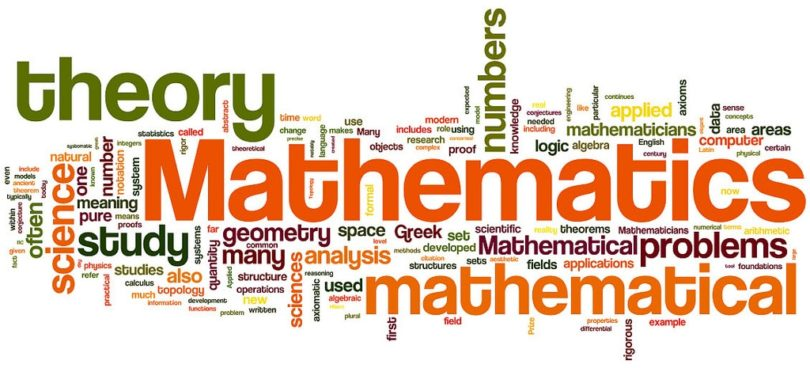Engineering Entrance Sample Papers

JCECE Objective Mathematics QuestionsThis JCECE Practice sample paper is based on JCECE syllabus and consist 34 questions, you can download all 34 questions in PDF format using link below last question.

Question: The length of subtangent to the curve x2y2 = a4 at the point (–a, a) is
(a) 3a
(b) 2a
(c) a
(d) 4a
Ans:- (c)

Question: The sides AB, BC, CA of a triangle ABC have respectively 3, 4 and  5 points lying on them. The number of triangles that can be constructed using these points as vertices is
(a) 205
(b) 220
(c) 210
(d) None of these
Ans:- (a)

Question: If x1, x2, x3 as well as y1, y2, y3 are in G.P. with the same common ratio, then the points (x1, y1), (x2, y2) and (x3, y3)
(a) Lie on a straight line
(b) Lie on an ellipse
(c) Lie on a circle
(d) Are vertices of a triangle
Ans:- (a)

Question: If the pair of straight lines xy – x – y + 1 = 0 and the line ax + 2y – 3 = 0 are concurrent, then a =
(a)  – 1
(b) 0
(c) 3
(d) 1
Ans:- (d)

Question: To expand (1 + 2x)–1/2 as an infinite series, the range of x should be
(a) [–1/2, 1/2]
(b) (–1/2, 1/2)
(c) [–2, 2]
(d) (–2, 2)
Ans:- (b)

Question: Two uniform solid spheres composed of the same material and having their radii 6 cm and 3 cm respectively are firmly united. The distance of the centre of gravity of the whole body from the centre of the larger sphere is
(a) 1 cm
(b) 3 cm
(c) 2 cm
(d) 4 cm
Ans:- (a)

Question: Coordinates of the vertices of a quadrilateral are (2, – 1),  (0, 2), (2, 3) and (4, 0). The angle between its diagonals will be
(a) 90o
(b) 0o
(c) tan–1 (2)
(d) tan–1 (1/2)
Ans:- (c)

Question: A man falls vertically under gravity with a box of mass ‘m’ on his head. Then the reaction force between his head and the box is
(a) mg
(b) 2 mg
(c) 0
(d) 1.5 mg
Ans:- (c)

Question: If the length of the tangents drawn from the point (1, 2) to the circles x2 + y2 + x + y – 4 = 0 and  3x2 + 3y2 – x – y + k = 0 be in the ratio 4 : 3, then k =
(a) 7/2
(b) 21/ 2
(c) – 21/ 4
(d) 7/ 4
Ans:- (c)

Question: The class marks of a distribution are 6,10, 14, 18, 22, 26, 30 then the class size is
(a) 4
(b) 2
(c) 5
(d) 8
Ans:- (a)

Question: The value of c so that for all real x, the vectors cxi + 6j + 3k, xi + 2j + 2cxk make an obtuse angle are
(a) c < 0
(b) 0 < c < 4/3
(c) –4/3 < c < 0
(d) c > 0
Ans:- (c)

Question: The maximum value of objective function in the above question is
(a) 100
(b) 92
(c) 95
(d) 94
Ans:- (c)

Question: Let E and F be two independent events. The probability that both E and F happens is 1/12 and the probability that neither E nor F happens is ½, then
(a) P(E) = 1/3, P(F) = ¼
(b) P(E) = ½, P(F) = 1/6
(c) P(E) = 1/6, P(F) = ½
(d) None of these
Ans:- (a)

Question: The control unit and arithmetic logic unit  is called
(a) Arithmetic Logic Unit (ALU)
(b) Central Processing Unit (CPU)
(c) Memory Unit
(d) Input Unit
Ans:- (b)

Question: The equation x3 – 3x + 4 = 0 has only one real root. What is its first approximate value as obtained by the method of false position in (–3, –2)
(a) – 2.125
(b) 2.125
(c) – 2.812
(d) 2.812
Ans:- (a)

Question: Let E = {1,2,3,4} and F = {1,2} . Then the number of onto functions from E to F is
(a) 14
(b) 16
(c) 12
(d) 8
Ans:- (a)

Related: JCECE Complete Syllabus

Question: If A is the set of even natural numbers less than 8 and B is the set of prime numbers less than 7, then the number of relations from A to B is
(a) 29
(b) 92
(c) 32
(d) 29 – 1
Ans:- (a)